# PostgreSQL 高级SQL(一)分组集

07/13 13:12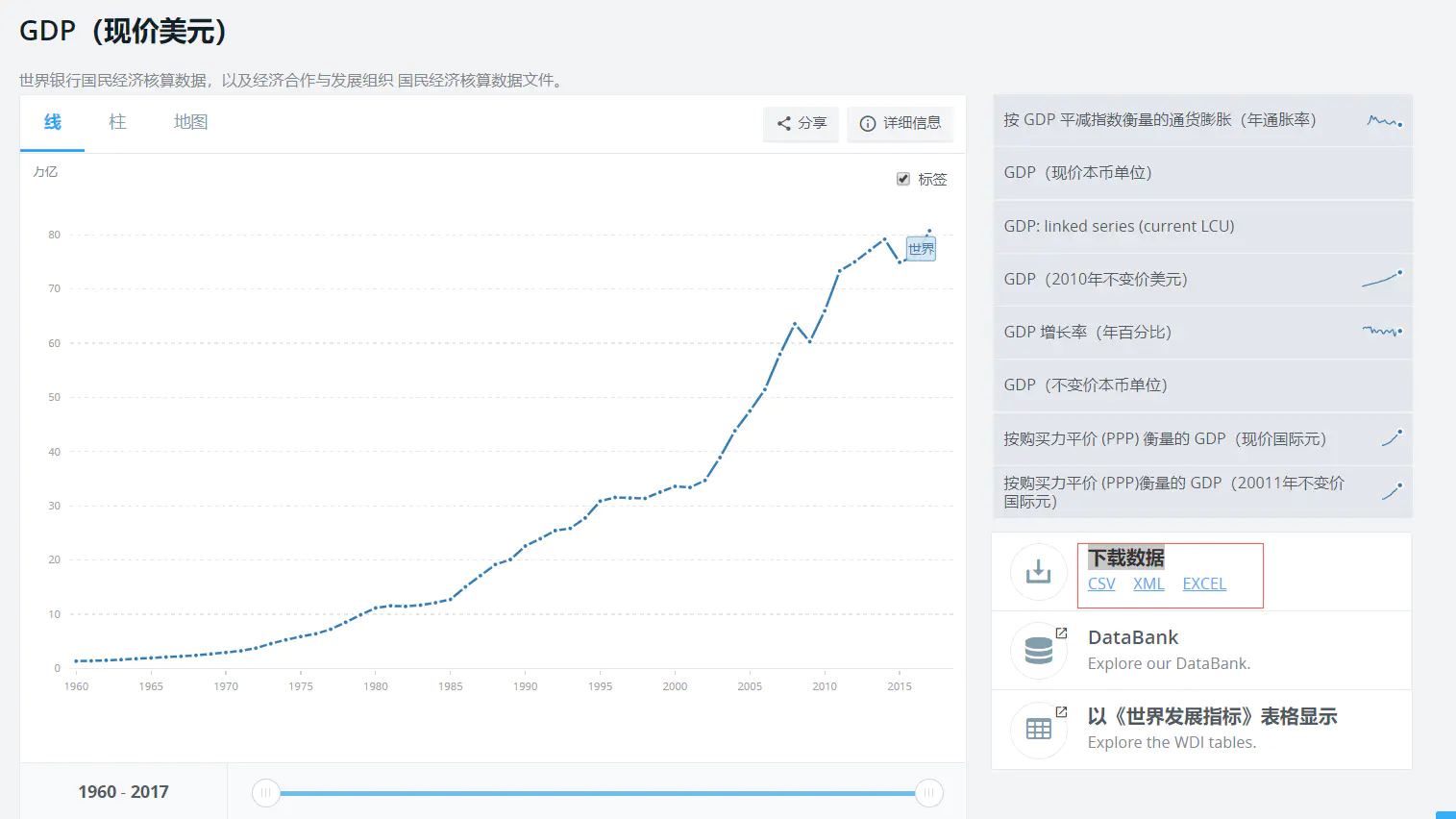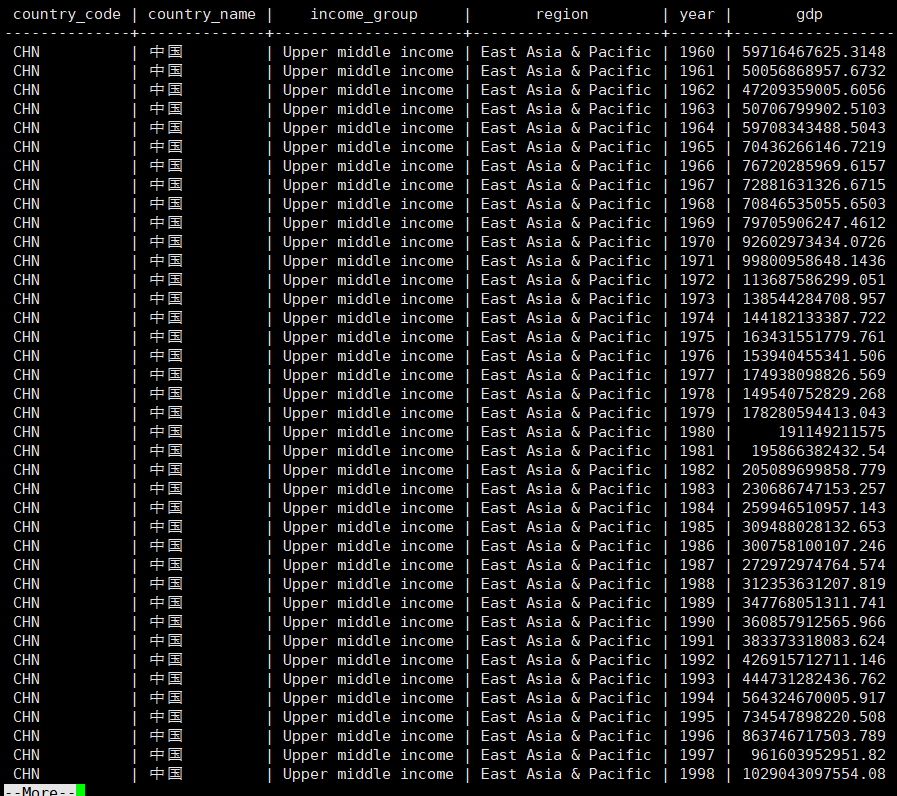1、如果我们想看一看世界各个大洲(区域)2017年的GDP平均值的话我们可以这样写：
``````SELECT
region,
round( AVG ( gdp ), 0 )
FROM
country_gdp_year_final
WHERE
YEAR = '2017'
GROUP BY
region
ORDER BY
2 DESC;``````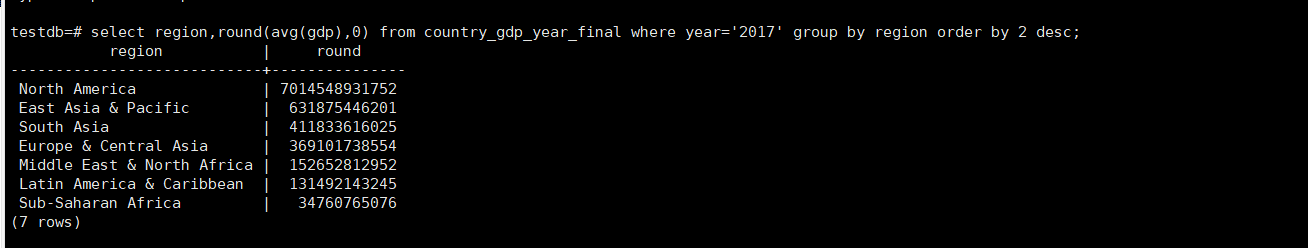group by非聚合函数可以很容易让我们看到2017年世界GDP均值最高地区是：北美洲，GDP最低地区是：撒哈拉以南非洲，group by 子句将很多行变成每组一行显示，

2、如果我们想要上面的报表带有全世界各国GDP平均值的话我们大部分人可能会出下面类似的SQL:

``````( SELECT
region,
round( AVG ( gdp ), 0 ) AS sumCount
FROM
country_gdp_year_final
WHERE
YEAR = '2017'
GROUP BY
region
ORDER BY
2 DESC
) UNION ALL
SELECT NULL AS
region,
sumCount
FROM
( SELECT round( SUM ( gdp ) / COUNT ( 1 ), 0 ) AS sumCount FROM country_gdp_year_final WHERE YEAR = '2017' ) AS P``````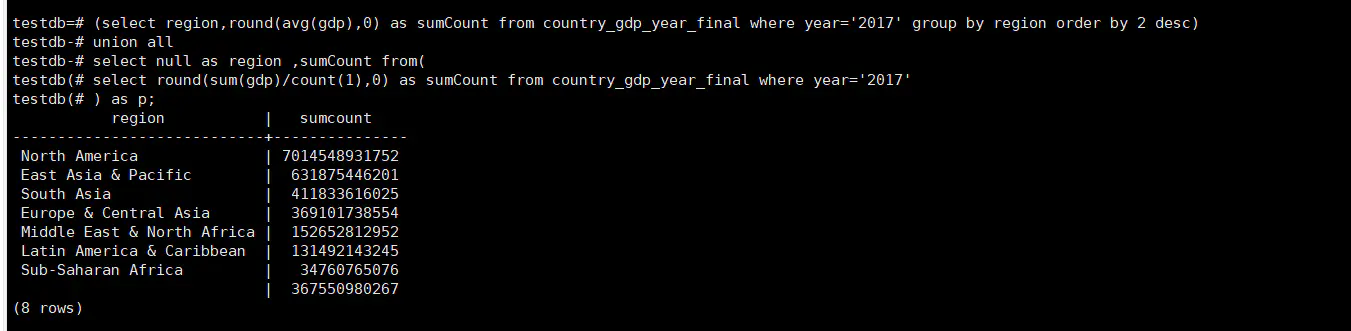``````SELECT
region,
round( AVG ( gdp ), 0 ) AS sumCount
FROM
country_gdp_year_final
WHERE
YEAR = '2017'
GROUP BY
ROLLUP ( region )
ORDER BY
2 DESC；``````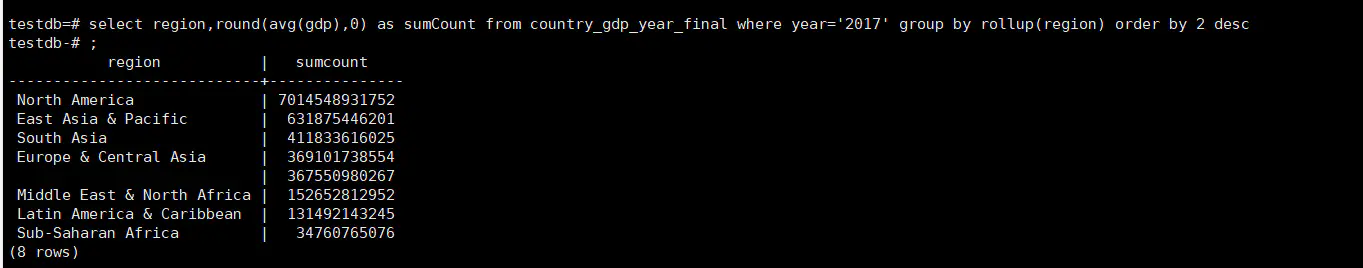group by rollup(column) 除了计算column字段分组下的聚合结果以外，还会计算整体的聚合结果，本例就是计算世界2017年GDP所有国平均值(这些国家有着一个共同的region叫做：世界)，无需运行俩个查询；

rollup也可以通过不止一列的方式来使用，比如计算中日德法美六国的1960-2018年年均GDP：

``````SELECT
region,
country_name,
round( AVG ( gdp ), 0 )
FROM
country_gdp_year_final
WHERE
country_code IN ( 'CHN', 'JPN', 'USA', 'DEU', 'CAN', 'FRA' )
GROUP BY
ROLLUP ( region, country_name );``````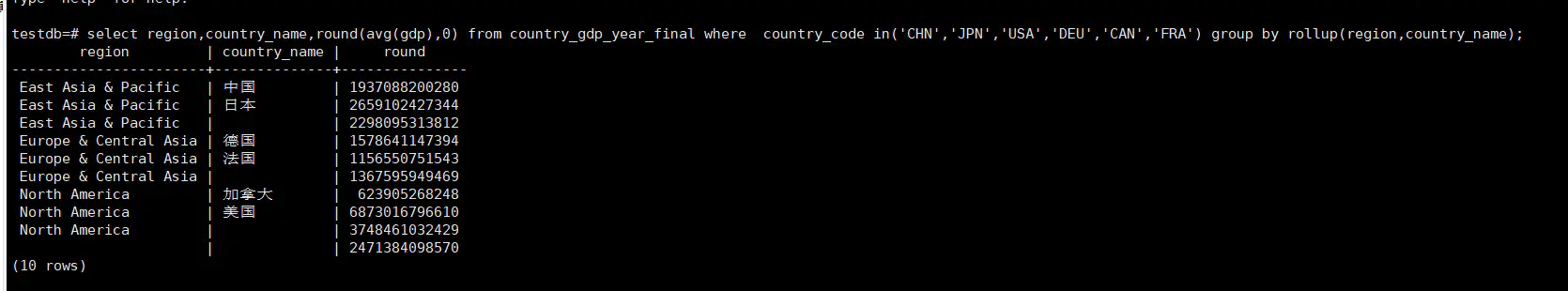3、group by cube子句

``````SELECT
region,
country_name,
round( AVG ( gdp ), 0 )
FROM
country_gdp_year_final
WHERE
country_code IN ( 'CHN', 'JPN', 'USA', 'DEU', 'CAN', 'FRA' )
GROUP BY
CUBE ( region, country_name );``````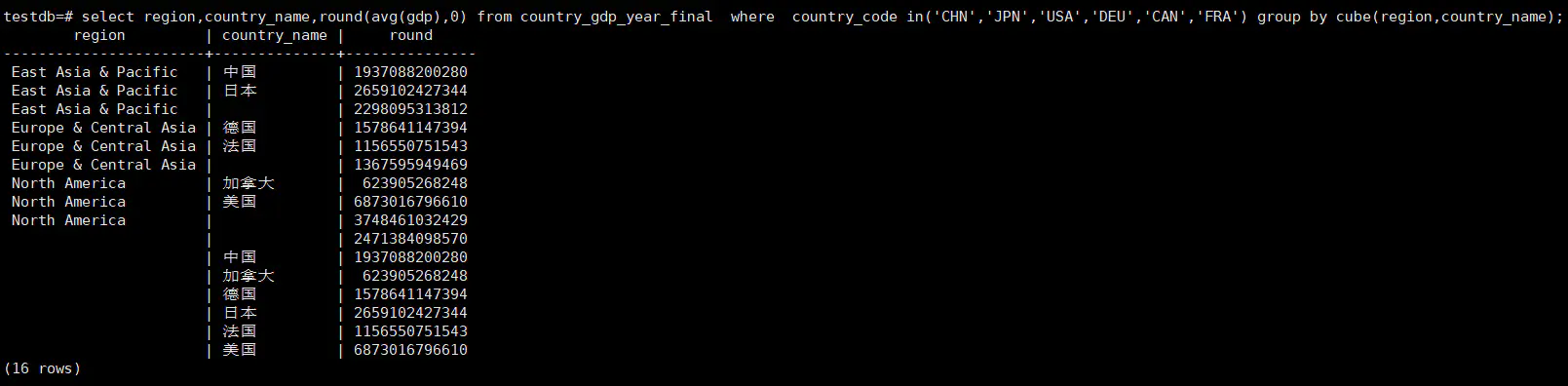cube:在英文里面是是立方体的意思，上面的句子group by cube(region,country_name)比group by rollup(region,country_name)多出了六行数据：

group by country_name 的六行，从上面的数据我们可以知道cube(region,country_name)的总行数=group by region,country_name+group by region+group by country_name+总体均值，其整体思想是抽取各个层面的聚合集结果。

4、group by grouping sets子句

``````SELECT
region,
country_name,
round( AVG ( gdp ), 0 )
FROM
country_gdp_year_final
WHERE
country_code IN ( 'CHN', 'JPN', 'USA', 'DEU', 'CAN', 'FRA' )
GROUP BY
GROUPING SETS ( ( ), region, country_name, ( region, country_name ) );``````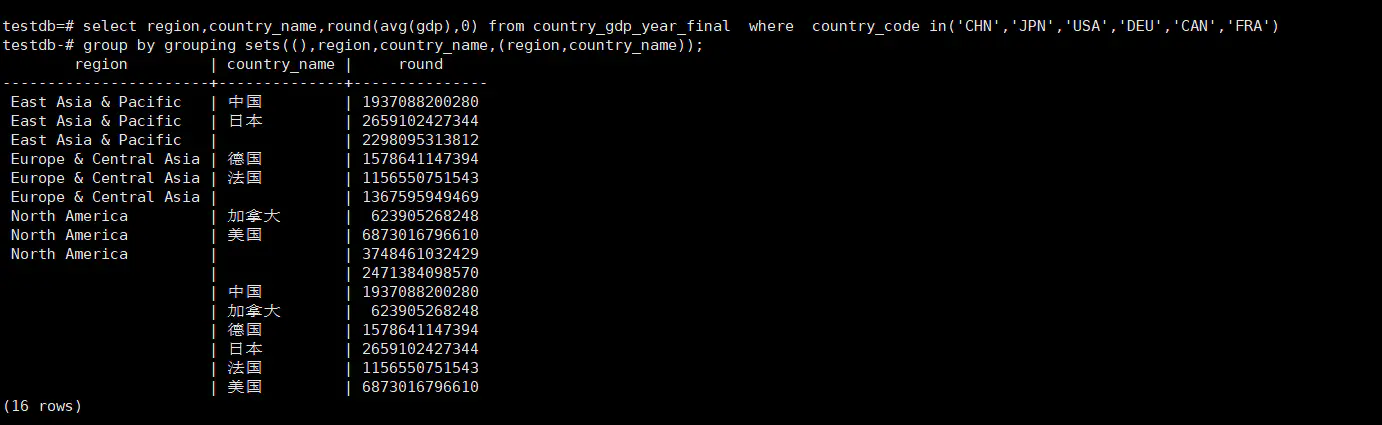A、

``````SELECT
region,
country_name,
round( AVG ( gdp ), 0 )
FROM
country_gdp_year_final
WHERE
country_code IN ( 'CHN', 'JPN', 'USA', 'DEU', 'CAN', 'FRA' )
GROUP BY
GROUPING SETS ( ( region, country_name ) );``````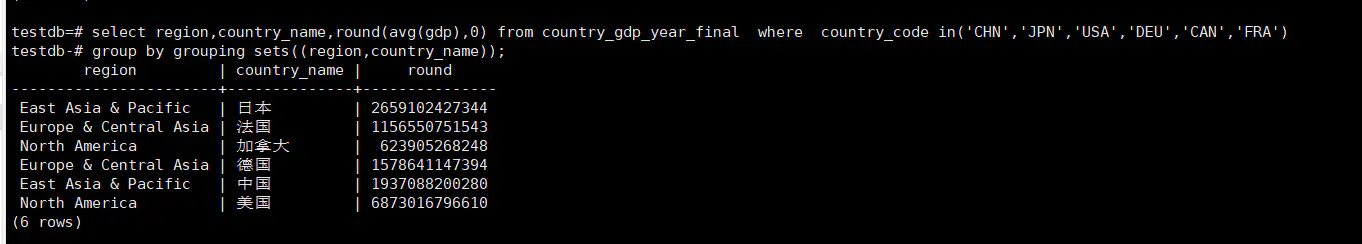B、

``````SELECT
region,
country_name,
round( AVG ( gdp ), 0 )
FROM
country_gdp_year_final
WHERE
country_code IN ( 'CHN', 'JPN', 'USA', 'DEU', 'CAN', 'FRA' )
GROUP BY
GROUPING SETS ( ( ), region, country_name );``````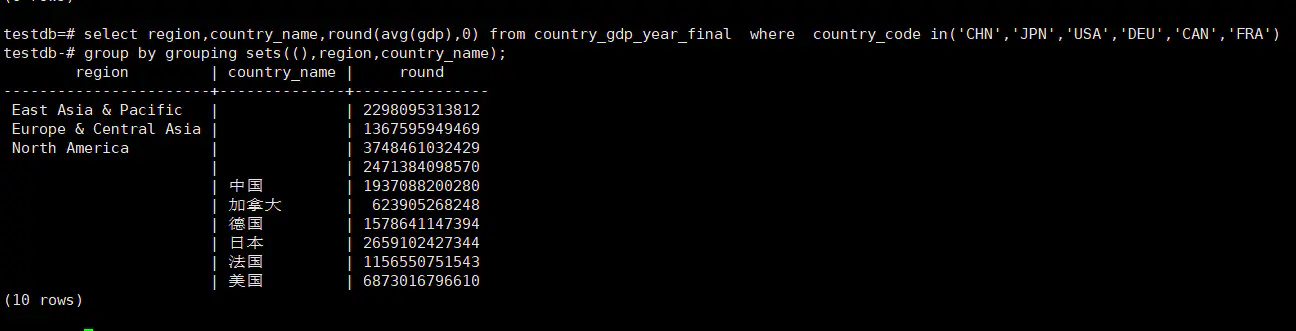C、

``````SELECT
region,
country_name,
round( AVG ( gdp ), 0 )
FROM
country_gdp_year_final
WHERE
country_code IN ( 'CHN', 'JPN', 'USA', 'DEU', 'CAN', 'FRA' )
GROUP BY
GROUPING SETS ( region, country_name );``````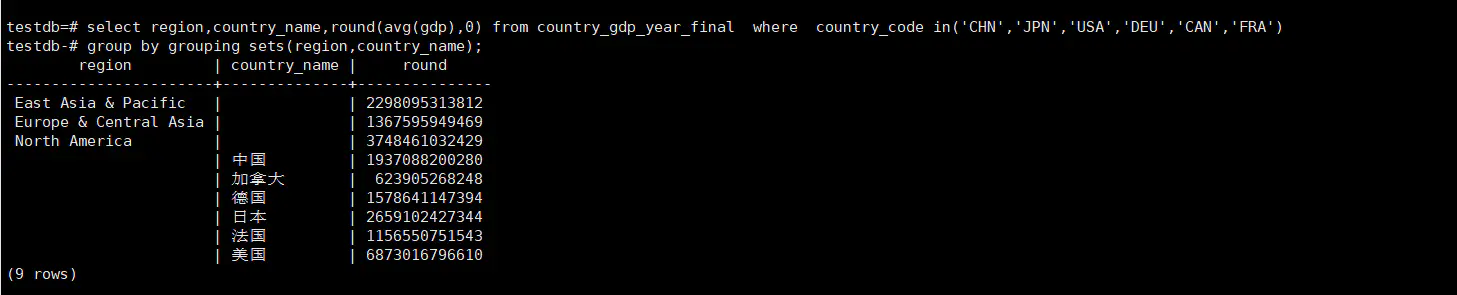0
0 收藏

### 作者的其它热门文章0 评论
0 收藏
0